Test: Context Free Language- 2

# Test: Context Free Language- 2

Test Description

## 15 Questions MCQ Test GATE Computer Science Engineering(CSE) 2023 Mock Test Series | Test: Context Free Language- 2

Test: Context Free Language- 2 for Computer Science Engineering (CSE) 2023 is part of GATE Computer Science Engineering(CSE) 2023 Mock Test Series preparation. The Test: Context Free Language- 2 questions and answers have been prepared according to the Computer Science Engineering (CSE) exam syllabus.The Test: Context Free Language- 2 MCQs are made for Computer Science Engineering (CSE) 2023 Exam. Find important definitions, questions, notes, meanings, examples, exercises, MCQs and online tests for Test: Context Free Language- 2 below.
Solutions of Test: Context Free Language- 2 questions in English are available as part of our GATE Computer Science Engineering(CSE) 2023 Mock Test Series for Computer Science Engineering (CSE) & Test: Context Free Language- 2 solutions in Hindi for GATE Computer Science Engineering(CSE) 2023 Mock Test Series course. Download more important topics, notes, lectures and mock test series for Computer Science Engineering (CSE) Exam by signing up for free. Attempt Test: Context Free Language- 2 | 15 questions in 45 minutes | Mock test for Computer Science Engineering (CSE) preparation | Free important questions MCQ to study GATE Computer Science Engineering(CSE) 2023 Mock Test Series for Computer Science Engineering (CSE) Exam | Download free PDF with solutions
 1 Crore+ students have signed up on EduRev. Have you?
Test: Context Free Language- 2 - Question 1

### Which of the following statements is true?

Detailed Solution for Test: Context Free Language- 2 - Question 1

(A) is wrong as a language can be context free even if it is being accepted by non deterministic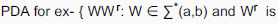reverse}

(C) and (D) not always true as Context free languages are not closed under Complement and Intersection.

Test: Context Free Language- 2 - Question 2

### Let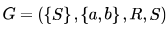be a context free grammar where the rule set R is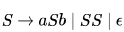Which of the following statements is true?

Detailed Solution for Test: Context Free Language- 2 - Question 2

It will be easy to analyze the problem if we replace terminal a and b by ( and ) respectively.

S →(S) | SS | ε

L(G) = balanced parentheses [each left parenthesis has a matching right parenthesis and are well nested ]

example () , ()(), (()), (()()()).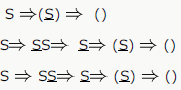String () can be derived by above three way each having different derivation tree.

So G is Ambiguous
b) Concatenation of two balance parenthesis will be balanced also . eq. x = (()) y= () xy= (())().
c) We can design Deterministic PDA for L. put left parenthesis (only) in stack and pop with right parenthesis.
d) We cannot design DFA for L because we need a stack to match left parenthesis with right parenthesis.
only option C is true.

Test: Context Free Language- 2 - Question 3

### Consider the languages: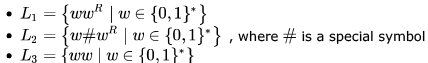Which one of the following is TRUE?

Test: Context Free Language- 2 - Question 4

Let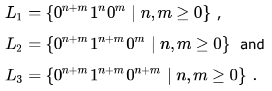Which of these languages are NOT context free?

Detailed Solution for Test: Context Free Language- 2 - Question 4

L1 is context-free. We count the number of 0's and check if the remaining number of 1's followed by 0's count to the initial
number of 0's.

L2 is not context-free. Here the number of 0's and the following 1's must be same, which can be checked using a PDA. But
after that we must also ensure that the following number of 0's must be less than the previous count of 0's and 1's
(otherwise n < 0, which violates the condition for acceptance) and we cannot do these two checks using a single PDA.

L3 is again not context-free as it is nothing but equal number of 0's followed by equal number of 1's followed by equal
number of 0's.

Test: Context Free Language- 2 - Question 5

In the context-free grammar below, S is the start symbol, a and b are terminals, and ϵ denotes the empty string.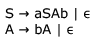The grammar generates the language

Detailed Solution for Test: Context Free Language- 2 - Question 5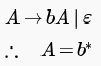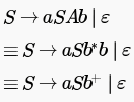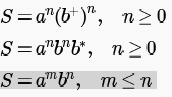Test: Context Free Language- 2 - Question 6

In the context-free grammar below, S is the start symbol, a and b are terminals, and ϵ denotes the empty string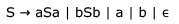Which of the following strings is NOT generated by the grammar?

Detailed Solution for Test: Context Free Language- 2 - Question 6

L(G) = PALINDROME
baba does not belong to palindrome , so B is the answer

Test: Context Free Language- 2 - Question 7

The two grammars given below generate a language over the alphabet {x, y, z}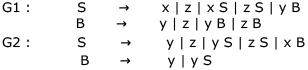Which one of the following choices describes the properties satisfied by the strings in these languages?

Detailed Solution for Test: Context Free Language- 2 - Question 7

from Above grammar
Regular expression for G1: (x+z)+ + (x+z)*y(y+z)+

Regular expression for G2 :(y+z+xy)+

Test: Context Free Language- 2 - Question 8

Consider the grammar given below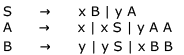Consider the following strings.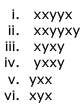Which of the above strings are generated by the grammar ?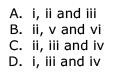Detailed Solution for Test: Context Free Language- 2 - Question 8

Above grammar is for equal no of x and y

from Non-terminal S -> xB

=>xy [as B->y one y for one x]

S->xB
=> xxBB [as B ->yBB one B result in one y for one x ]

S->xB
=>xyS [as B->yS one y for one x and start again]

Test: Context Free Language- 2 - Question 9

Consider the following grammars. Names representing terminals have been specified in capital letters.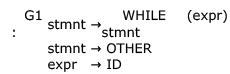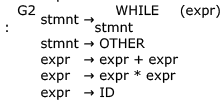Which one of the following statements is true?

Detailed Solution for Test: Context Free Language- 2 - Question 9

Regular grammar is either right linear or left linear. A left linear grammar is one in which there is at most 1 non-terminal on the right side of any production, and it appears at the left most position. Similarly, in right linear grammar non-terminal appears at the right most position.

Here, we can write a right linear grammar for G1 as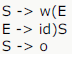(w - WHILE, o - OTHER)

So, L(G1) is regular.

Now for G2 also we can write a right linear grammar: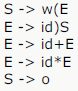making its language regular.

So, both G1 and G2 have an equivalent regular grammar. But as such both these grammars are neither right linear nor left
linear and hence not a regular grammar. So, D must be the answer.

Test: Context Free Language- 2 - Question 10

A CFG G is given with the following productions where S is the start symbol, A is a non-terminal and a and b are terminals.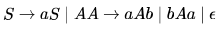Which of the following strings is generated by the grammar above?

Detailed Solution for Test: Context Free Language- 2 - Question 10

S→ aS
S→ aA
S→ aaAb
S→ aabAab
S→ aabbAaab
S→ aabbaab

Test: Context Free Language- 2 - Question 11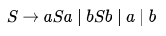The language generated by the above grammar over the alphabet  is the set of

Detailed Solution for Test: Context Free Language- 2 - Question 11

string generated by this language is a,b,aba,bab,aabaa,.....all this strings are odd length palindromes

Test: Context Free Language- 2 - Question 12

Which of the following languages are context-free?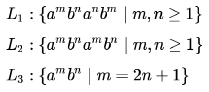Detailed Solution for Test: Context Free Language- 2 - Question 12

first check for L1. now look a^m&b^m and a^n&b^n must b comparable using one stack for CFL. now take a stack push all a^m in to the stack then push all b^n in to stack now a^n is coming so pop b^n for each a^n by this b^n and a^n will b comparable. now we have left only a^m in stack and b^m is coming so pop a^m for each b^m by which we can compare a^m to b^m ..we conclude that we are comparing this L1 using a single stack so this is CFG.

now for L2.this can not be done in to a single stack because m and n are not comparable we can not find when to push or pop so this is CSL.

now for L3.push all a's into stack and pop 2a 's for every b and at last we left with a single a .
bcz here aaaaabb is a valid string where m=2n+1 and n=2.So realized using single stack hence L3 is CFG.

Test: Context Free Language- 2 - Question 13

Consider the following context-free grammar over the alphabet ∑  = {a,b,c } with S as the start symbol: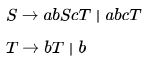Which one of the following represents the language generated by the above grammar?

Detailed Solution for Test: Context Free Language- 2 - Question 13

Consider the 1st production S-> abScT
This production generates equal number of (ab)'s and c's but after each c there is T which goes to T->bT

so with each c there can be one or more b's (one because of production T->b and more because of T->bT)

and these b's are independent.

For example

ababcbbcbb is the part of the langugage
and ababcbbbbbbcbb is also the part of the language so we rule out A and C both say equal number of b's after each c

In option D equal number of (ab)'s and c's is not satisfied . The only option that satisfies these 2 conditions is B

number of (ab)'s and c's is not satisfied . The only option that satisfies these 2 conditions is B

people who says this will be the string at any case

S-> abcT

-> abcb

no problem for i B in this case also here cmn=cm1 means n value 1 thats it

and remember in B c is not coming eternally.it is coming until m value reaches n of b..right there

.if m=n=1 then cb stops

Test: Context Free Language- 2 - Question 14

If G is a grammar with productions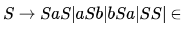where S is the start variable, then which one of the following strings is not generated by G ?

Test: Context Free Language- 2 - Question 15

Consider a CFG with the following productions.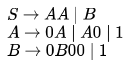S is the start symbol, A and B are non-terminals and 0 and 1 are the terminals. The language generated by this grammar is

Detailed Solution for Test: Context Free Language- 2 - Question 15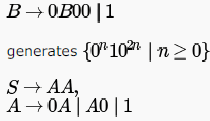generates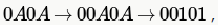which is there in only B and D choices. D is not the answer as "00" is not generated by the given grammar. So, only option left is B and if we see carefully, non-terminal B is generating the second part of B choice and AA is generating the first part.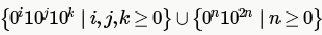## GATE Computer Science Engineering(CSE) 2023 Mock Test Series

150 docs|215 tests
 Use Code STAYHOME200 and get INR 200 additional OFF Use Coupon Code
Information about Test: Context Free Language- 2 Page
In this test you can find the Exam questions for Test: Context Free Language- 2 solved & explained in the simplest way possible. Besides giving Questions and answers for Test: Context Free Language- 2, EduRev gives you an ample number of Online tests for practice

## GATE Computer Science Engineering(CSE) 2023 Mock Test Series

150 docs|215 tests